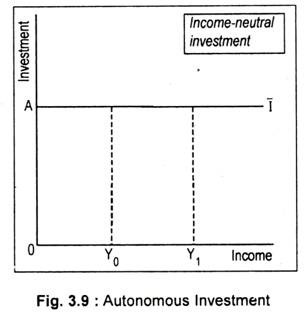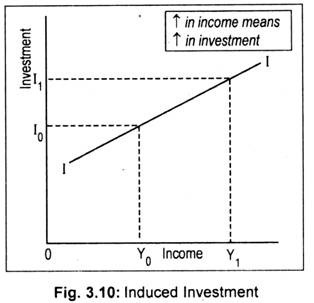# Investment Types: Autonomous and Induced Investment

The two types of investments are discussed below:

#### (i) Autonomous Investment:

Investment may be autonomous and induced. Usually, investment decision is governed by output and/or the rate of interest.

If investment does not depend either on income/output or the rate of interest, then such investment is called autonomous investment. Thus, autonomous investment is independent of the level of income.It is evident from Fig. 3.9 that, whatever the level of income, the level of autonomous investment has been fixed at OA. To describe this type of investment we have put a bar sign over the head of the curve I. Thus, autonomous investment, as per Fig. 3.9, is income-neutral.

#### (ii) Induced Investment:

Investment that is dependent on the level of income or on the rate of interest is called induced investment. Investment that would respond to a change in national income or in the rate of interest is called induced investment. Fig. 3.10 shows that, as national income rises from OY0 to 0Y1, (induced) investment increases from OI0 to OI1. Thus, investment that is income-elastic is called induced investment.That is,

I = f(Y)

The slope of the investment line II is the marginal propensity to invest (MPl). MPl is the ratio of change in investment to the change in income. Or the ratio of increase in investment (A I) to an increase in income (A Y) is called MPl, i.e.,

MPL = ∆l/∆Y

Keynes believed that interest rate and the expectation of future profitability of investment projects are the two main determinants of investment expenditures in the short run. Investment is inversely related to the level of interest rate, i.e., I = f(r)